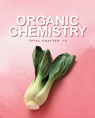Click to Chat

1800-1023-196

+91-120-4616500

CART 0

• 0

MY CART (5)

Use Coupon: CART20 and get 20% off on all online Study Material

ITEM
DETAILS
MRP
DISCOUNT
FINAL PRICE
Total Price: Rs.

There are no items in this cart.
Continue Shopping
`        Consider the two first order reactions X → Y and Y → X for which the rate constants k1 and k2 are 4.0 ×10^3 s^(−1) and 2.0×10^3 s^(−1) respectively. One mole of X and three moles of Y are allowed to react and the concentrations of X and Y are measured as a function of time and plotted.  The correct figure showing the variation of the concentrations [X] and [Y] with time and the correct equilibrium concentrations [X]eq and [Y]eq of X and Y are (Figures are not drawn to scale.)`
11 months ago

```							 Consider the rxn X ->YThe eqilibrium constant keq=k1/k2 =2=[Y]/[X]Let the no. Of moles of y reacted to give x be aSo, 2=(3-a)/(1+a)       a=1/3 Therefore, at equilibrium[X]=4/3[Y]=8/3 Hence, option (B) is correct
```
9 months ago
Think You Can Provide A Better Answer ?

## Other Related Questions on Organic Chemistry

View all Questions »### Course Features

• 731 Video Lectures
• Revision Notes
• Previous Year Papers
• Mind Map
• Study Planner
• NCERT Solutions
• Discussion Forum
• Test paper with Video Solution### Course Features

• 70 Video Lectures
• Revision Notes
• Test paper with Video Solution
• Mind Map
• Study Planner
• NCERT Solutions
• Discussion Forum
• Previous Year Exam Questions